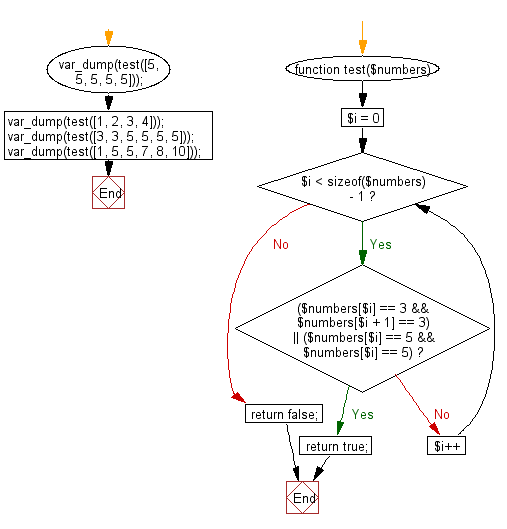﻿ PHP Exercises: Check if an array of integers contains a 3 next to a 3 or a 5 next to a 5 or both - w3resource# PHP Exercises: Check if an array of integers contains a 3 next to a 3 or a 5 next to a 5 or both

## PHP Basic Algorithm: Exercise-116 with Solution

Write a PHP program to check if an array of integers contains a 3 next to a 3 or a 5 next to a 5 or both.

Sample Solution:

PHP Code :

``````<?php
function test(\$numbers)
{

for (\$i = 0; \$i < sizeof(\$numbers) - 1; \$i++)
if ((\$numbers[\$i] == 3 && \$numbers[\$i + 1] == 3) || (\$numbers[\$i] == 5 && \$numbers[\$i] == 5))
return true;
return false;
}

var_dump(test([5, 5, 5, 5, 5]));
var_dump(test([1, 2, 3, 4]));
var_dump(test([3, 3, 5, 5, 5, 5]));
var_dump(test([1, 5, 5, 7, 8, 10]));
``````

Sample Output:

```bool(true)
bool(false)
bool(true)
bool(true)
```

Flowchart:PHP Code Editor:

What is the difficulty level of this exercise?

﻿

## PHP: Tips of the Day

\$_REQUEST: This SuperGlobal Variable is used to collect data submitted by a HTML Form

Example:

This code save in a php file and run in the browser.

```<!DOCTYPE html>
<html>
<body>

<form method="post" action="<?php echo \$_SERVER['PHP_SELF'];?>">
NAME: <input type="text" name="user">
<button type="submit">SUBMIT</button>
</form>
<?php
if (\$_SERVER["REQUEST_METHOD"] == "POST") {
\$name = htmlspecialchars(\$_REQUEST['user']);
if(empty(\$name)){
echo "Name is empty";
} else {
echo \$name;
}
}
?>
</body>
</html>
```

Output:

```Owen
```